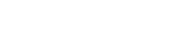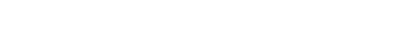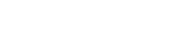## Questions

1. In this question, show all the steps in your calculations, giving the answer at each stage. Use logarithms correct to 4 decimal places, to evaluate;2. The table below shows monthly income tax rates
 Monthly taxable pay in KE Rate of the tax (Kshs/E) 1-342 2 343-684 3 685-1026 4 1027-1368 5 1369-1710 6 1710 and above 7

Mr. Kamau who is a civil servant earns a Monthly salary of Kshs.20000 and is provided with a house at a nominal rent of Kshs.700 per month
1. Taxable pay is the employee’s salary plus 15% less nominal rent. Calculate Mr.Kamau’s taxable pay
2. Calculate the total tax Mr. Kamau pays
3. Mr. Kamau is entitled to a personal relief of Kshs.600 per month. What was his net tax.
4. Mr. Kamau has the following deductions made on his pay;
Loan repayment of Kshs.2100 per month
NSSF Kshs.200 per month
WCPS calculated at 2 % of monthly salary
Calculate Mr. Kipchokes net pay
3. A man bought a matatu at Kshs.400,000 in January 1999. It depreciated at a rate of 16% per annum. If he valued it six months, calculate its value in January 2003
4. The table shows corresponding values of x and y for a certain curve;Using 3 strips and mid-ordinate rule estimate the area between the curve, x-axis, the lines x =1 and x =2.2
5. Evaluate without using a calculator or mathematical tables.
Log 32 + log 128 − log 729
Log 32 + log 2 − log 27
6. Find the value of x that satisfies the equation:-
log (x+5) = log 4 − log(x+2)
7. Find the least number of terms for which the sum of the GP 100 + 200 + 400 + ……………….. exceeds 3100.
8. A two digit number is formed from the first four prime numbers.
1. Draw the table to show the possible outcomes, if each number can be used only once.
2. Calculate the probability that a number chosen from the digit numbers is an even number
9. Find the gradient of a line joining the centre of a circle whose equation is x² + y² − 6² = 3 – 4y and a point P(6,7) outside the circle.
10. A lady invests shs.10,000 in an account which pays 16% interest p.a. The interest is compounded quarterly. Find the amount in the account after 1½ hrs
11. Use logarithm tables to evaluate12. Without using logarithms or calculator evaluate:
2log10 5 − 3log10 2 + log10 32
13. Evaluate without using tables or calculators.
Log (3x + 8) − 3 log2 = log x − 4

1.2.
1. monthly taxable pay;
15% of monthly salary = 15/100 x 20000
= kshs.3000
Monthly pay = Kshs.(20000 + 3000 − 700)
= Kshs. 22300
In Kenya pounds = 22300/20
= KE 1115
2. Total tax payable (Gross tax)
1 − 342_________ 342x2 = Kshs.684
343 − 684 _______ 342 x3=Kshs.1026
685 − 1026 ______ 342 x4= Kshs.1368
1027 − 1368 _____ 89x5 = Kshs.445
Total tax = Kshs.3523
3. Net tax
= Gross tax – relief
= Kshs.(3523 – 600) = Kshs.2923
4. Net pay;
= Kshs.20000 – (2923 + 2100 + 200 + 2/100 x 20000)
= Kshs. (20000 – 5623) = Kshs.14377
3. 6 month depreciation rate = 8%
Number of periods = 8
400,000 (1 – 0.08)8 = 205288
4. Mid ordinate
Area = 1.2 (6.2 + 4.3 + 2.6)
= 15.72
5.6. Log (x+5) = log(4)/(x+2)
x + 5 = 4/(x + 2)
(x+5) (x+2) = 4
x2+ 2x + 5X + 10 = 4
x2+ 7x + 6 = 0
x2+ 6x + x + 6 =0
x(x+6) + 1(x+6) = 0
(x +1) (x +6) = 0
x = -1 x = -6
7. a = 100
r = 200 = 2
100
a (rn -1) Sn
r - 1
100 (2n – 1) 3,100
2 – 1
2n – 1 > 31
2n >32
2n >25
n >5
n = 6
1. 2      3       5         7
2      32     52       72
3      23     53       73
5      25     35       75
7      27     37       57
2. P(E) = 4/16
= ¼
8.  x2 + y2 – 6x = 3 – 4y
x2– 6x + (-6/2)2 + y2 + 4y + (4/2)2 = 3 + (-6/2)2 + (4/2)2
(x – 3)2 (y + 2)2 = 3 + 9 = 4
(x – 3)2 (y + 2)2 = 16
C (3, -2)
Gradient y = 7 - -2 = 3
∆x     6 – 3
9.  A = P(1 + r/100 )n
= 10000 (1 + 4/100)6
= 10000(1.04)6
= 12653.19 (12,653)
10.11.12.#### Download Further Logarithms Questions and Answers - Form 3 Topical Mathematics.

• ✔ To read offline at any time.
• ✔ To Print at your convenience
• ✔ Share Easily with Friends / Students

Read 9139 times Last modified on Wednesday, 10 February 2021 07:01
.
Subscribe now

access all the content at an affordable rate
or
Buy any individual paper or notes as a pdf via MPESA
and get it sent to you via WhatsApp